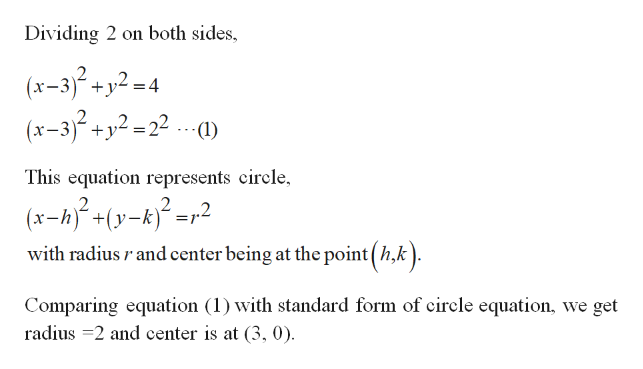# Write in standard form with graphing:2(x-3)^2+2y^2=8

Question
4 views

Write in standard form with graphing:

2(x-3)^2+2y^2=8

check_circle

Step 1

Provide the equation in standard form and graph.

Step 2

Reduce the equation to...help_outlineImage TranscriptioncloseDividing 2 on both sides, (x-32+y2-4 (x-32+y2=22 (1) This equation represents circle, (x-hy-k2 _ with radius rand center being at the point h,k) Comparing equation (1) with standard form of circle equation, we get radius 2 and center is at (3, 0) fullscreen

### Want to see the full answer?

See Solution

#### Want to see this answer and more?

Solutions are written by subject experts who are available 24/7. Questions are typically answered within 1 hour.*

See Solution
*Response times may vary by subject and question.
Tagged in

### Other